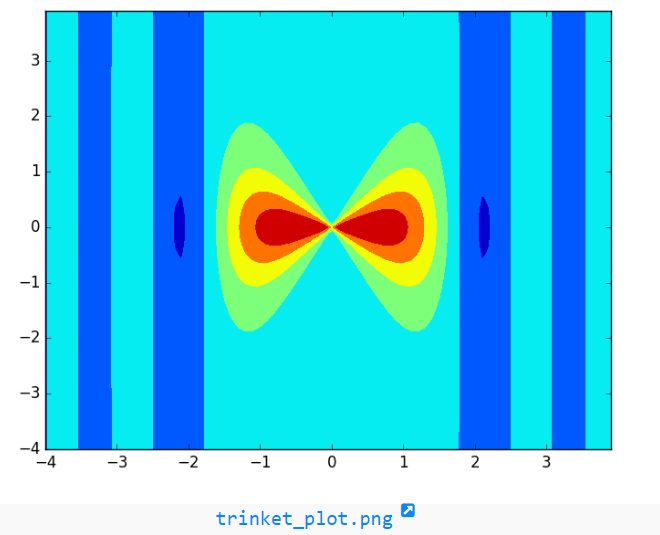# NumPy array creation: meshgrid() function

## numpy.meshgrid() function

The meshgrid() function is used to get coordinate matrices from coordinate vectors.
Make N-D coordinate arrays for vectorized evaluations of N-D scalar/vector fields over N-D grids, given one-dimensional coordinate arrays x1, x2,…, xn.

Syntax:

`numpy.meshgrid(*xi, **kwargs)`

Version: 1.15.0

Parameter:

Name Description Required /
Optional
x1, x2,…, xn 1-D arrays representing the coordinates of a grid.
indexing Cartesian (‘xy’, default) or matrix (‘ij’) indexing of output. See Notes for more details.
New in version 1.7.0.
optional
sparse If True a sparse grid is returned in order to conserve memory. Default is False.
New in version 1.7.0.
optional
endpoint If True, stop is the last sample. Otherwise, it is not included. Default is True. optional
copy If False, a view into the original arrays are returned in order to conserve memory. Default is True. Please note that sparse=False, copy=False will likely return non-contiguous arrays. Furthermore, more than one element of a broadcast array may refer to a single memory location. If you need to write to the arrays, make copies first.
New in version 1.7.0.
optional

Return value:

X1, X2,…, XN : ndarray - For vectors x1, x2,…, ‘xn’ with lengths Ni=len(xi) , return (N1, N2, N3,...Nn) shaped arrays if indexing=’ij’ or (N2, N1, N3,...Nn) shaped arrays if indexing=’xy’ with the elements of xi repeated to fill the matrix along the first dimension for x1, the second for x2 and so on.

Example-1: NumPy.meshgrid() function

``````>>> import numpy as np
>>> ab, ac = (4, 2)
>>> b = np.linspace(0, 1, ab)
>>> c = np.linspace(0, 1, ac)
>>> bd, cd = np.meshgrid(b, c)
>>> bd
array([[ 0.        ,  0.33333333,  0.66666667,  1.        ],
[ 0.        ,  0.33333333,  0.66666667,  1.        ]])
>>> cd
array([[ 0.,  0.,  0.,  0.],
[ 1.,  1.,  1.,  1.]])
>>> bd, cd = np.meshgrid(b, c, sparse=True) #make sparse output arrays
>>> bd
array([[ 0.        ,  0.33333333,  0.66666667,  1.        ]])
>>> cd
array([[ 0.],
[ 1.]])
``````

Example - 2: NumPy.meshgrid() function

``````>>> import numpy as np
>>> import matplotlib.pyplot as plt
>>> import numpy as np
>>> a = np.arange(-4, 4, 0.1)
>>> b = np.arange(-4, 4, 0.1)
>>> aa, bb = np.meshgrid(a, b, sparse=True)
>>> c = np.sin(aa**2) / (aa**2 + bb**2)
>>> m = plt.contourf(a,b,c)
>>> plt.show()
``````

numpy.logspace.plot showPython - NumPy Code Editor:

Previous: geomspace()
Next: mgrid()

﻿